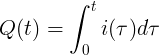# Electric charge

### What is electric charge?

Electric charge generates electric field. The electric charge influence other electric charges with electric force and influenced by the other charges with the same force in the opposite direction.

There are 2 types of electric charge:

#### Positive charge (+)

Positive charge has more protons than electrons (Np>Ne).

Positive charge is denoted with plus (+) sign.

The positive charge attracts other negative charges and repels other positive charges.

The positive charge is attracted by other negative charges and repelled by other positive charges.

#### Negative charge (-)

Negative charge has more electrons than protons (Ne>Np).

Negative charge is denoted with minus (-) sign.

Negative charge attracts other positive charges and repels other negative charges.

The negative charge is attracted by other positive charges and repelled by other negative charges.

#### Electric force (F) direction according to charge type

q1/q2 charges Force on q1 charge Force on q2 charge
- / - ←⊝ ⊝→ repletion
+ / + ←⊕ ⊕→ repletion
- / + ⊝→ ←⊕ attraction
+ / - ⊕→ ←⊝ attraction

#### Charge of elementary particles

Particle Charge (C) Charge (e)
Electron 1.602×10-19 C

-e

Proton 1.602×10-19 C

+e

Neutron 0 C 0

### Coulomb unit

The electric charge is measured with the unit of Coulomb [C].

One coulomb has the charge of 6.242×1018 electrons:

1C = 6.242×1018 e

### Electric charge calculation

When electric current flows for a specified time, we can calculate the charge:

#### Constant current

Q = I t

Q is the electric charge, measured in coulombs [C].

I is the current, measured in amperes [A].

t is the time period, measured in seconds [s].

#### Momentary currentQ is the electric charge, measured in coulombs [C].

i(t) is the momentary current, measured in amperes [A].

t is the time period, measured in seconds [s].

Currently, we have around 5612 calculators, conversion tables and usefull online tools and software features for students, teaching and teachers, designers and simply for everyone.